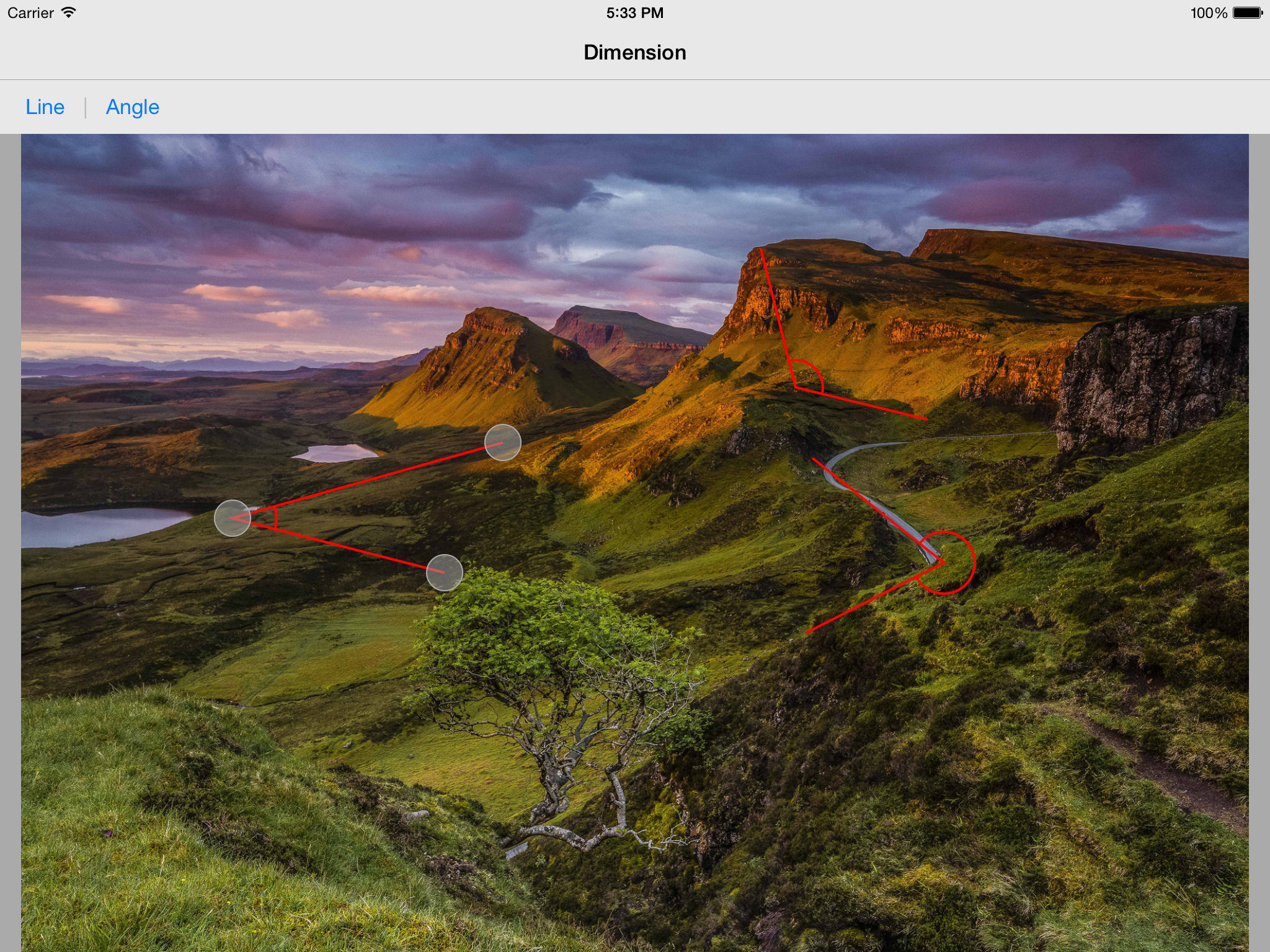# Core Graphics – Drawing an angle dimension from 3 arbitrary points.

For a recent project, I needed to have the ability for a user to draw an angle dimension on top of a picture. They can drag the 3 points and the dimension lines should update in real time. I needed to draw two lines and an arc between the two lines. Drawing the two lines is simple, but drawing the arc took a little bit of thinking.

At first I was calculating the inscribed angle using the law of cosines, then trying to determine the starting angle. The end angle would be the starting angle + the inscribed angle. Big mistake. This is complicated and has too many edge cases for each quadrant a starting or ending point is located in. Then I tried the handy atan2 function, which previously proved very useful in game design, and worked perfectly. In this case, imagine a line of 3 points, where each point can be moved and the angle between the two segments are shown.

Below is my drawing code to achieve this:

```- (void)drawRect:(CGRect)rect
{
[super drawRect:rect];

CGFloat lx = self.leftEndpoint.center.x;// first point along edge
CGFloat ly = self.leftEndpoint.center.y;
CGFloat mx = self.middleEndpoint.center.x;// middle pt
CGFloat my = self.middleEndpoint.center.y;
CGFloat rx = self.rightEndpoint.center.x;// other point along edge
CGFloat ry = self.rightEndpoint.center.y;

CGContextRef context = UIGraphicsGetCurrentContext();
CGContextSetStrokeColorWithColor(context, [self.lineColor CGColor]);

// thickness
CGContextSetLineWidth(context, 2.0f);

// starting pt
CGContextMoveToPoint(context, lx, ly);
// middle pt
// ending pt
// draw path, make sure to call this here to reset the stoke path points
CGContextStrokePath(context);

// angle arc
// distances
CGFloat lmDist = sqrt(pow(lx - mx, 2) + pow(ly - my, 2));
CGFloat mrDist = sqrt(pow(rx - mx, 2) + pow(ry - my, 2));
// angles
CGFloat startAngle = atan2(ry - my, rx - mx);// right to middle
CGFloat endAngle = atan2(ly - my, lx - mx);// left to middle
CGFloat radius = 0.20 * fmin(lmDist, mrDist);// 20% of min distance along lines
int clockwise = YES;